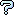All about flooble | fun stuff | Get a free chatterbox | Free JavaScript | Avatarsperplexus dot infoTruncated Cone (Posted on 2004-01-23)If you have a truncated cone such that its upper base has a radius of a and the radius of its [larger] lower base is b, and a height h (between bases), how could you figure out its surface area using geometric reasoning?

 See The Solution Submitted by Gamer Rating: 2.5000 (4 votes)Comments: ( Back to comment list | You must be logged in to post comments.)Geometric Interpretation of Simpler Formula?| Comment 8 of 10 |(In reply to re(3): general equation: by Richard)

To show

pi*b*sqrt(b^2+((h*b/(b-a))^2)-pi*a*sqrt(a^2+((h*a/(b-a))^2)

= pi*(a+b)*sqrt((b-a)^2+h^2)

write

b^2 + (h^2*b^2)/(b-a)^2 = b^2*((b-a)^2+h^2)/(b-a)^2 and

a^2 + (h^2*a^2)/(b-a)^2 = a^2*((b-a)^2+h^2)/(b-a)^2. Hence

pi*b*sqrt(b^2+((h*b/(b-a))^2)-pi*a*sqrt(a^2+((h*a/(b-a))^2)

= (pi* (b^2-a^2)/(b-a))*sqrt((b-a)^2+h^2)

= pi*(a+b)*sqrt((b-a)^2+h^2).

Thus the area of the curved part of the truncated cone can be expressed in the simpler form

pi*(a+b)*sqrt((b-a)^2+h^2) given by Ady TZIDON as well as in the form

pi*b*sqrt(b^2+((h*b/(b-a))^2)-pi*a*sqrt(a^2+((h*a/(b-a))^2)

geometrically obtained by removing a small full cone from a larger one. Is there a simple geometric interpretation for the simpler formula?
 Posted by Richard on 2004-01-26 19:06:43Please log in:

 Search: Search body:
Forums (0)